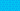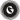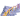PricingResources

# What is forward rate?Written by

If you’re considering making an investment in Treasury bills, it’s imperative to have a solid understanding of the forward rate equation. Put simply, the forward rate formula can give you greater insight into the theoretical price of a financial transaction at some point in the future. Get a little more information about forward rate and the forward rate calculation formula with our in-depth guide.

## Understanding the forward rate equation

Forward rate is the theoretical yield on a bond that will occur in the future (in most cases, several months or years from the time of the calculation). Yield is a term referring to the return on the bond buyer’s investment. Generally, forward rate is used when discussing the purchase of T-bills, or Treasury bills. When you’re calculating the forward rate equation, you can use one of the following methods:

• Yield curve – Essentially, this refers to the relationship between interest rates on government bonds at different levels of maturity. While it provides a clear insight into present-day bond prices and interest rates, the yield curve also enables you to predict future interest rates. In most cases, the yield curve is the best way to determine the forward rate.

• Spot rates – Spot rates can help you to identify the assumed yield on a zero-coupon T-bill. Spot rate is a less commonly used way to calculate the forward rate, although it can be effective.

## Difference between spot rate and forward rate

Although the definition of “spot rate” and forward rate” can differ depending on the market they’re used in, there’s a very clear difference between spot rate and forward rate that you’ll need to understand to get to grips with the forward rate equation. Essentially, spot rates refer to the current price or bond yield, whereas forward rates refer to the future price or bond yield. So, while spot rates help you understand how much it costs to make a financial transaction today, forward rates provide you with insight into how much it might cost to make a transaction at a later date.

## Forward rate formula

If you’re using the yield curve to calculate forward rate, there are three distinct forward rate formulas that you can use: simple rate, yearly compounded rate, and continuously compounded rate. However, we’re going to focus on the forward rate calculation formula for calculating from spot rates. Essentially, you can convert the spot rate to the forward rate using the following forward rate formula:

Where:

Let’s see a forward rate example to get a better sense of how this works in practice. Imagine that a three-year bond is yielding 9%, while a two-year bond is yielding 5%. Here’s how a forward rate calculation formula can help you get results:

For this forward rate example, 17.46% is the hypothetical forward rate of the investment.

## How is the forward rate calculation formula used?

Firstly, understanding how to use the forward rate formula can be highly beneficial for investors that are considering purchasing Treasury bills, as they can help you to choose the option with the highest potential profits. It’s also worth noting that forward rate equations are widely used in hedging and currency markets, so it’s a useful tool for anyone involved in these areas of finance.

## We can help

GoCardless helps you automate payment collection, cutting down on the amount of admin your team needs to deal with when chasing invoices. Find out how GoCardless can help you with ad hoc payments or recurring payments.Contact sales

Sales

Support